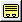# Mathematical Expressions

Make a property a function of other properties in your model by entering a mathematical expression in a text box. A mathematical expression functions like a cell formula in a spreadsheet. Press Enter to automatically evaluate the expression and display the computed value in the text box.

A mathematical expression can be constructed using any of MotionView’s math functions and operators. Entity variable names and property names are used to refer to properties of other entities.

Example mathematical expressions:

(p_1.x +p_2.x) / 2

b_powertrain.mass * 1.5

b_uca.l.cm.origin.z + ds_params.wb.value

You can access the various properties of an entity by using a period (.) and the name of the property. For instance, in the preceding example, we are accessing the following properties:

 p_1.x The x coordinate of point p_1. p_2.x The x coordinate of point p_2. b_powertrain.mass The mass of body b_powertrain. b_uca.l.cm.origin.z The z coordinate of the origin point of the center-of-mass-marker of body b_uca. ds_params.wb.value The value of the variable, wb, in dataset ds_params.

To refer to an entity in another system, you must include the name of the system and model in your reference. As with properties, system names are seperated from variable names using a period.

 model.sys_front.p_1.x The x coordinate of point p_1 in system sys_front in model model. sys_plane.sys_wing.sys_flap.j_hinge.body1.mass The mass of the body attached to joint j_hinge in the system sys_flap in the system sys_wing in the model sys_plane.

You can also use the Expression Builder to construct mathematical expressions. See the Use the Expression Builder topic for additional information.

## View the Variable Name of an Entity or System

1. From the Project Browser, select an entity or system.
The corresponding panel is displayed.
2. Click.
The "Entity" Info or System Info dialog is displayed that contains the entity or system variable name.